# How to create a calculator using assignments and expressions

Creating a calculator involves two steps.

1. Assignment
2. Expressions

### Assignment:

In the first step, you'll set a score or value for an answer using the assignment feature. The default assignment options are Number, Rating, and Opinion Scale, which allow you to take the answered value as a score.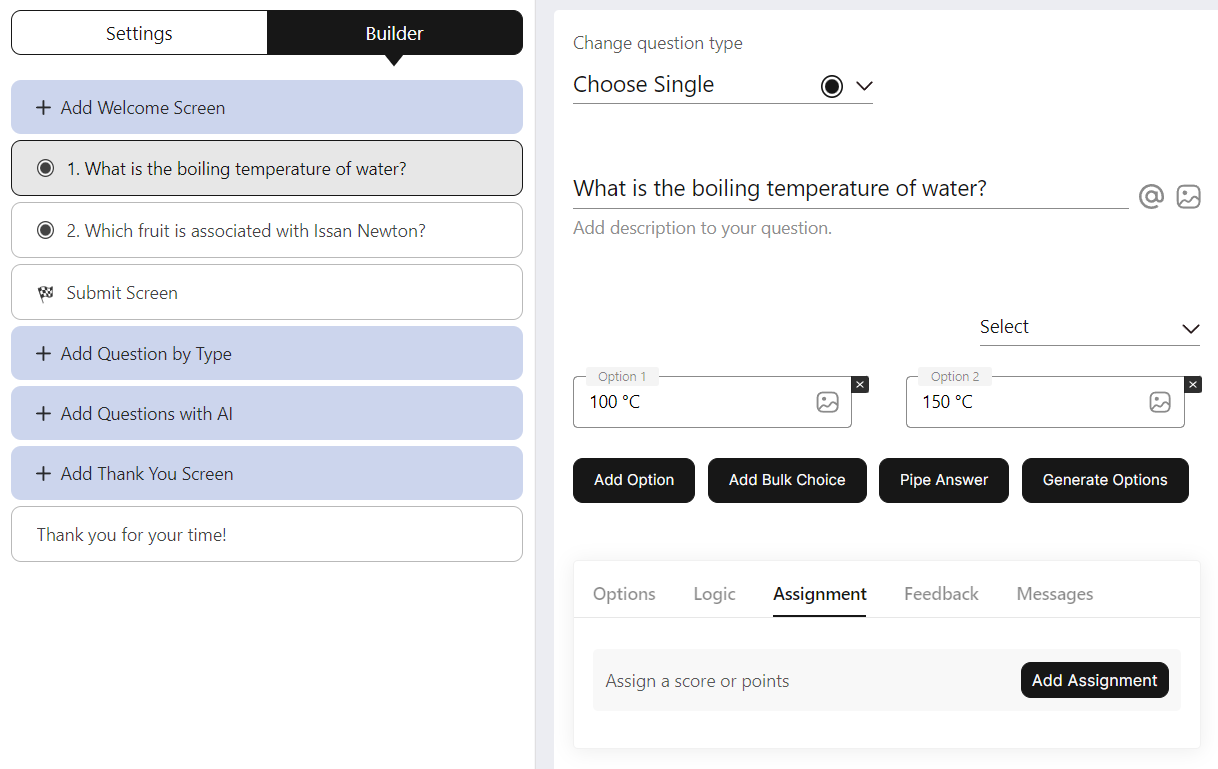When an assignment is added for a question, the scores are calculated and added to a default variable called 'Score' in the expressions section.

### Expressions:

Expressions are used to write formulas for calculations. You can access them in Settings -> Assignment & Formulas -> Numeric Expressions.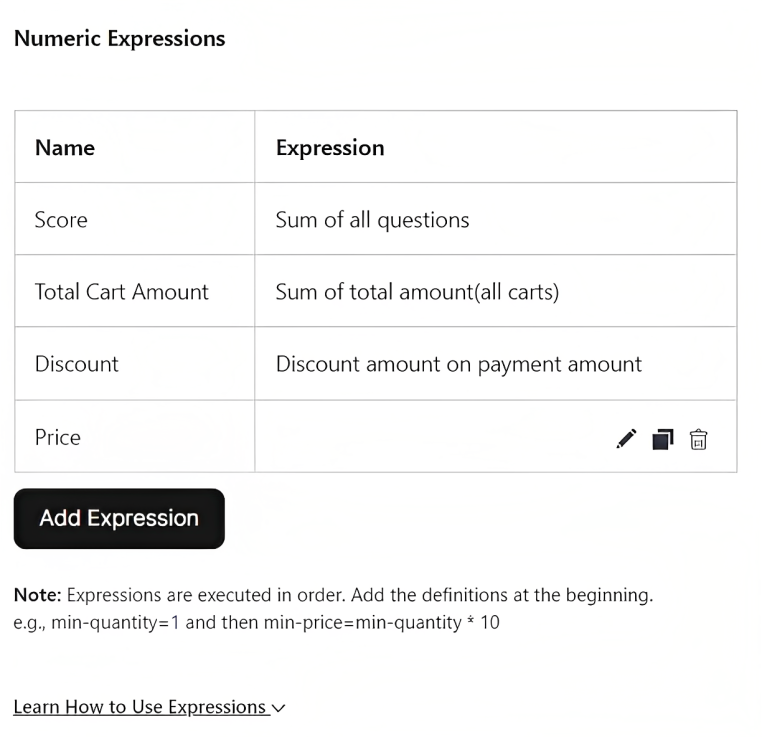BlockSurvey has a default expression called Score, which sums the scores of all questions. You can create your expressions and formulas based on your needs by clicking Add Expression and selecting questions and expressions using the @ symbol.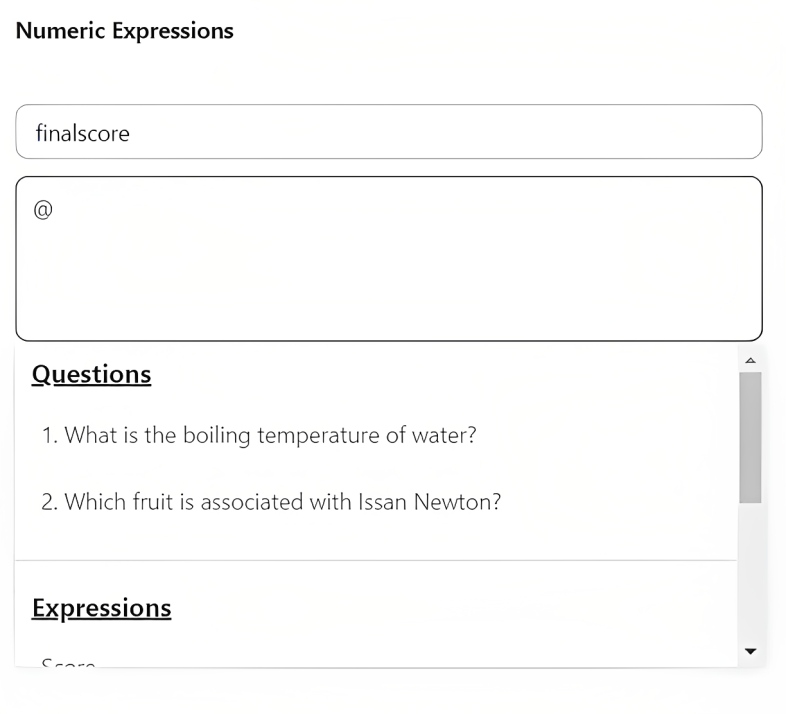All mathematical operations supported in Javascript are supported in Expressions. Refer following links for reference Operators, Comparisons, and Math.

### Example:

Now that we have understood the basics let's create a simple Return on Investment(ROI) Calculator

### What is the ROI?

Return on Investment ( ROI) is a financial metric commonly used to calculate an investment return likelihood. It's a ratio that compares an investment's gain or loss to its expense. It is useful in calculating future returns from a stand-alone investment compared to multi-investment returns.

ROI = (Net Return on Investment / Initial of Investment) X 100

Let's create questions to accept the Initial Investment and Final Investment to calculate and show Return on Investment.

### Assignment:

Question 1: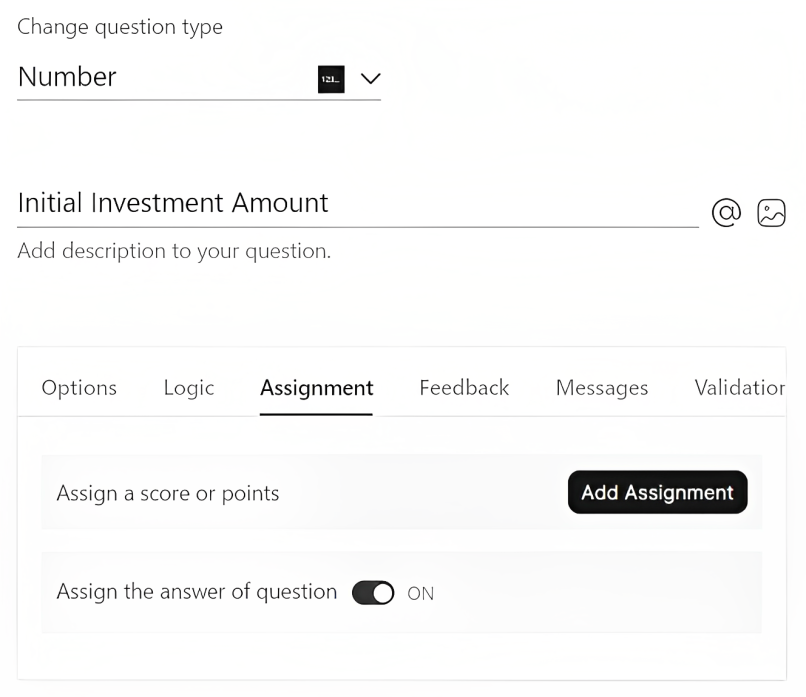Question 2: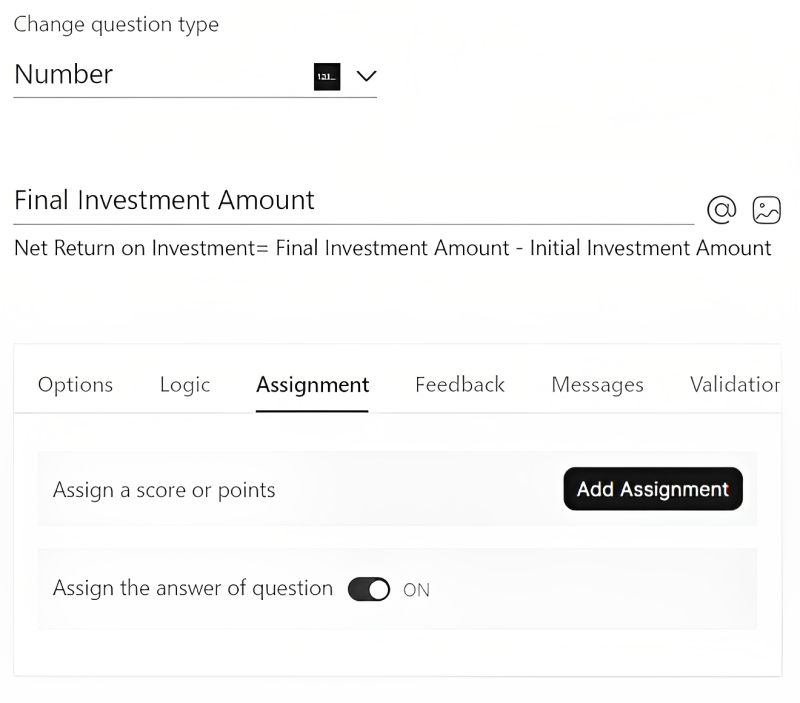ROI Question: You can see how @ has been used to select the expressions.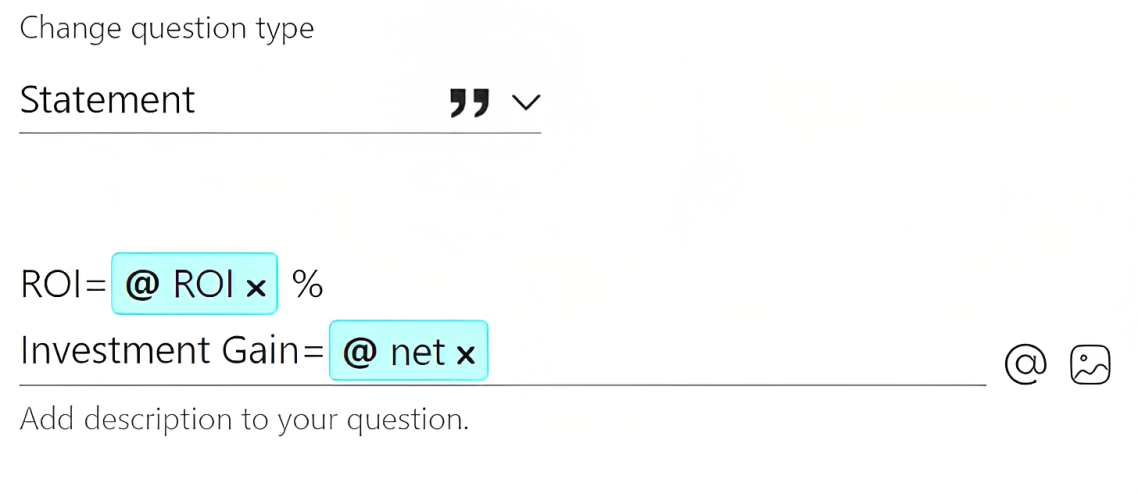#### Expressions:

You can observe the expression written for net return and how net return is later used in ROI expression.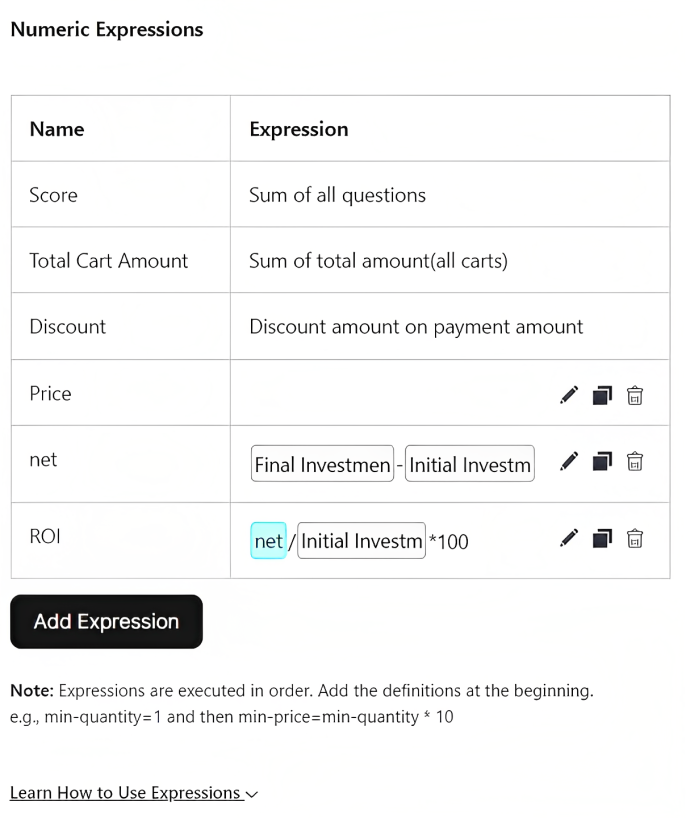Good luck with creating your calculator.

If you are still running into issues, contact us at [email protected] at any time.